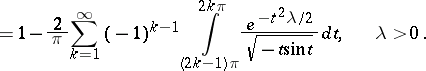Omega-squared distribution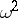-distribution

The probability distribution of the random variable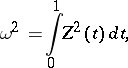whereis a conditional Wiener process (conditioned on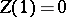). The characteristic function of the "omega-squared" distribution is expressed by the formula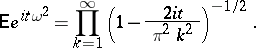In mathematical statistics, the "omega-squared" distribution is often found in the following circumstances. Letbe independent random variables, uniformly distributed on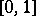, according to which an empirical distribution functionis constructed. In this case, the process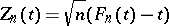converges weakly to a conditional Wiener process, from which it follows that